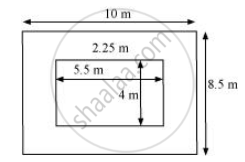Share

# A Verandahof Width 2.25 M is Constructed All Along Outside a Room Which is 5.5 M Long and 4 M Wide. Find: - CBSE Class 7 - Mathematics

ConceptConcept of Application of Conversion

#### Question

A verandah of width 2.25 m is constructed all along outside a room which is 5.5 m long and 4 m wide. Find:

1) the area of the verandah

2) the cost of cementing the floor of the verandah at the rate of Rs 200 per m2.

#### Solution1)

Length (l) of room = 5.5 m

Breadth (b) of room = 4 m

Area of room = l × b = 5.5 × 4 = 22 m2

From the figure, it can be observed that the new length and breadth of the room, when verandah is also included, are 10 m and 8.5 m respectively.

Area of the room including the verandah = 10 × 8.5 = 85 m2

Area of verandah = Area of the room including the verandah − Area of room

= 85 − 22 = 63 m2

2)

Cost of cementing 1 m2 area of the floor of the verandah = Rs 200

Cost of cementing 63 m2 area of the floor of the verandah = 200 × 63

= Rs 12600

Is there an error in this question or solution?

#### APPEARS IN

NCERT Solution for Mathematics for Class 7 (2018 to Current)
Chapter 11: Perimeter and Area
Ex. 11.40 | Q: 4 | Page no. 226
Solution A Verandahof Width 2.25 M is Constructed All Along Outside a Room Which is 5.5 M Long and 4 M Wide. Find: Concept: Concept of Application of Conversion.
S